Courses

# Test: Complex Numbers

## 30 Questions MCQ Test Mathematics (Maths) Class 11 | Test: Complex Numbers

Description
This mock test of Test: Complex Numbers for JEE helps you for every JEE entrance exam. This contains 30 Multiple Choice Questions for JEE Test: Complex Numbers (mcq) to study with solutions a complete question bank. The solved questions answers in this Test: Complex Numbers quiz give you a good mix of easy questions and tough questions. JEE students definitely take this Test: Complex Numbers exercise for a better result in the exam. You can find other Test: Complex Numbers extra questions, long questions & short questions for JEE on EduRev as well by searching above.
QUESTION: 1

### Number of values `p' for which the equation (p2 – 3p + 2) x2 – (p2 – 5p + 4)x + p – p2 = 0 possess more than two roots, is

Solution: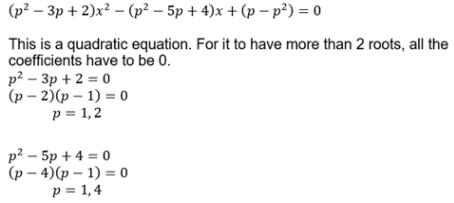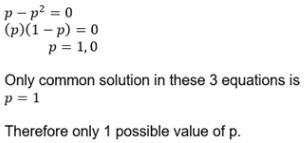QUESTION: 2

Solution:
QUESTION: 3

### If a, b are the roots of quadratic equation x2 + px + q = 0 and g, d are the roots of x2 + px – r = 0, then (a – g) . (a - d) is equal to

Solution:
QUESTION: 4

Two real numbers a & b are such that a + b = 3 & |a - b| = 4, then a & b are the roots of the quadratic equation

Solution: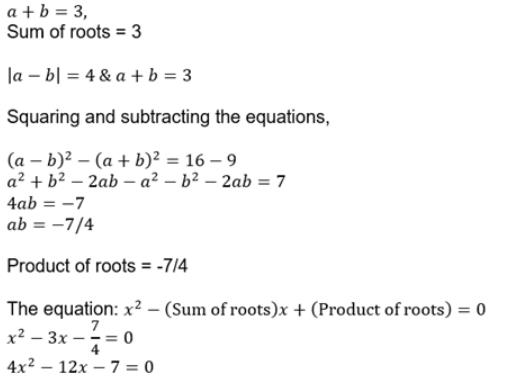QUESTION: 5

If a, b, c are integers and b2 = 4(ac + 5d2), d ∈ N, then roots of the equation ax2 + bx + c = 0 are

Solution:

b2 = 4(ac + 5d2)
b2 = 4ac + 20d2
b2 – 4ac = 20d2
D = 20d

As D > 0, roots of the equation ax2 + bx + c = 0 are real.

D1/2 = 2*(51/2) *d
D1/2 is irrational.

As a, b, c are integers, roots of the equation are irrational.

QUESTION: 6

Let a, b and c are real numbers such that 4a + 2b + c = 0 and ab > 0. Then the equation ax2 + bx + c = 0 has

Solution: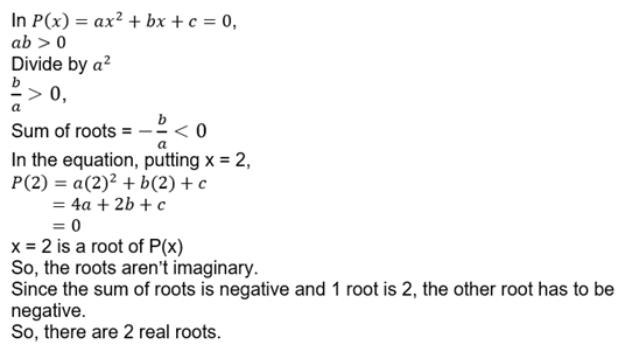QUESTION: 7

Which of the following graph represents expression f(x) = ax2 + bx + c (a < 0) when a > 0, b < 0 & c < 0 ?

Solution: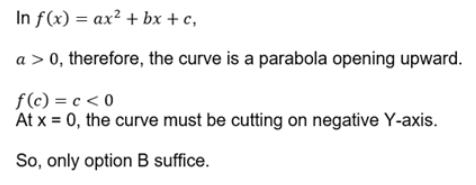QUESTION: 8

The expression y = ax2 + bx + c has always the same sign as of `a' if

Solution:

For the quadratic curve to always have the same sign, it must never cut the X-axis, i    i.e. it must not have any real roots.
So, b2 – 4ac < 0
4ac > b2

QUESTION: 9

If a, b ∈ R, a < 0 and the quadratic equation ax2 – bx + 1 = 0 has imaginary roots then a + b + 1 is

Solution:

The correct option is A.

Error in the question : a is not less than zero. It should be a0

We have D0

(-b)2-4a0a0

So the parabola formed is open upwards

f(-1)=a+b+10 as for negative values of x , y is always positive

QUESTION: 10

If a and b are the non–zero distinct roots of x2 + ax + b = 0, then the least value of x2 + ax + b is

Solution:
QUESTION: 11

If y = –2x2 – 6x + 9, then

Solution:
QUESTION: 12

The number of the integer solutions of x2 + 9 < (x + 3)2 < 8x + 25 is

Solution: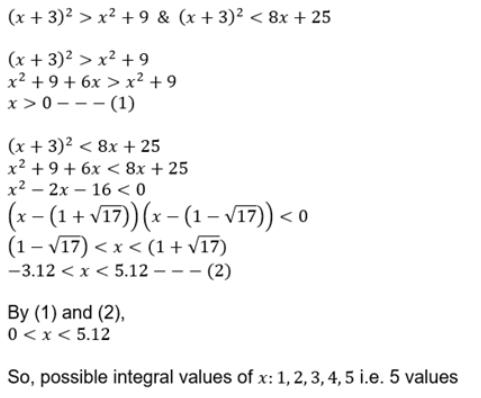QUESTION: 13

If both roots of the quadratic equation (2 – x) (x + 1) = p are distinct & positive, then p must lie in the interval

Solution: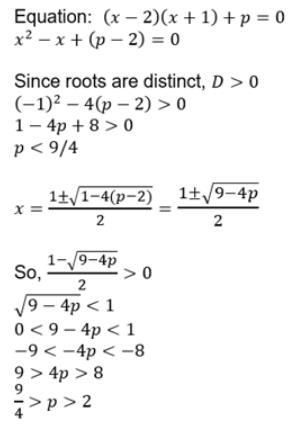QUESTION: 14

The equatiobn πx = –2x2 + 6x – 9 has

Solution:

The RHS of the expression has a<0 which means the graph will lie below the x-axis and πx lies above the x-axis.Therefore,no solution.

QUESTION: 15

Let a > 0, b > 0 and c > 0. Then both the roots of the equation ax2 + bx + c = 0

Solution: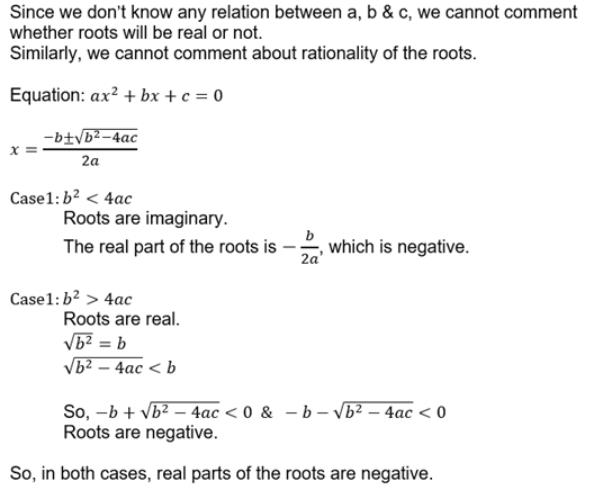QUESTION: 16

The set of all solutions of the inequality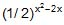< 1/4 contains the set

Solution: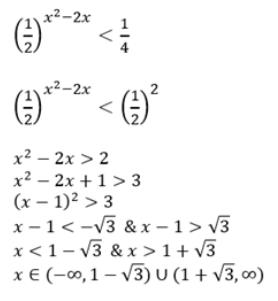QUESTION: 17

Consider y =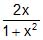, where x is real, then the range of expression y2 + y – 2 is

Solution: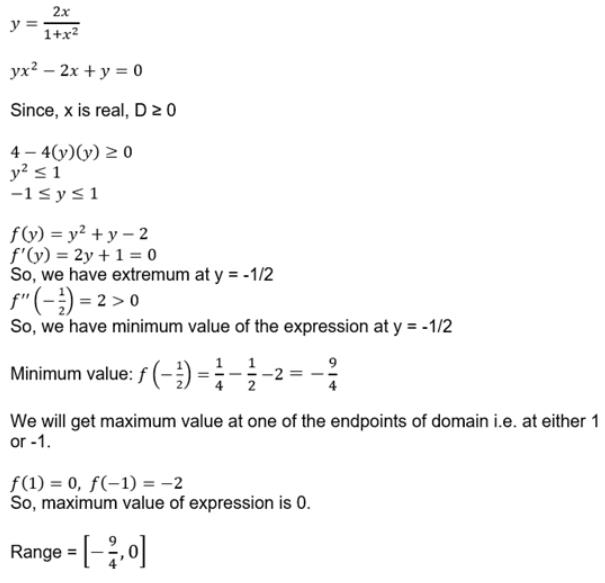QUESTION: 18

The values of k, for which the equation x2 + 2(k – 1) x + k + 5 = 0 possess atleast one positive root, are

Solution: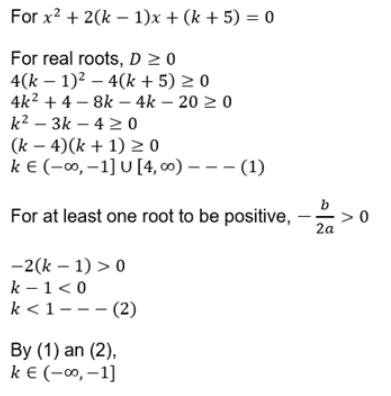QUESTION: 19

If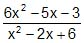> 4, then least and the highest values of 4x2 are

Solution: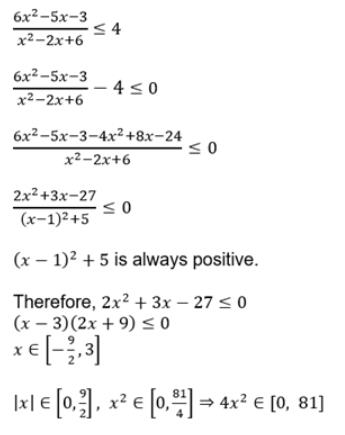QUESTION: 20

If coefficients of the equation ax2 + bx + c = 0, a < 0 are real and roots of the equation are non–real complex and a + c + b < 0, then

Solution:
QUESTION: 21

If the roots of the equation x2 + 2ax + b = 0 are real and distinct and they differ by at most 2m, then b lies in the interval

Solution:
QUESTION: 22

If the roots of the quadratic equation x2 + px + q = 0 are tan 30º and tan 15º respectively, then the value of 2 + q – p is

Solution:
QUESTION: 23

If (1 – p) is root of quadratic equation x2 + px + (1 – p) = 0, then its roots are

Solution:
QUESTION: 24

The values of x and y besides y can satisfy the equation (x, y ∈ real numbers) x2– xy + y2 – 4x – 4y + 16 = 0

Solution:
QUESTION: 25

For what value of a and b the equation x4 – 4x3 + ax2 + bx + 1 = 0 has four real positive roots ?

Solution:
QUESTION: 26

If a, b are roots of the equation ax2 + bx + c = 0, then the value of a3 + b3 is

Solution:
QUESTION: 27

If z1 = 2 + i, z2 = 1 + 3i, then Re ( z1 - z2) =

Solution:

The numbers in the questions are not very clear.
z1 = 2 + i
z2 = 1+3i
z1 – z2 = (2 – 1) + i (1 – 3)
= 1 – 2i

QUESTION: 28

If a, b are roots of the equation ax2 + bx + c = 0 then the equation whose roots are 2a + 3b and 3a + 2b is

Solution:
QUESTION: 29

If S is the set of all real x such that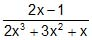is positive, then S contains

Solution:
QUESTION: 30

If the roots of the equation x2 - 5x + 16 = 0 are a, b and the roots of the equation x2 + px + q = 0 are (a2 + b2) and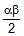, then-

Solution: